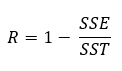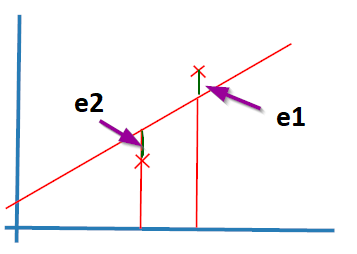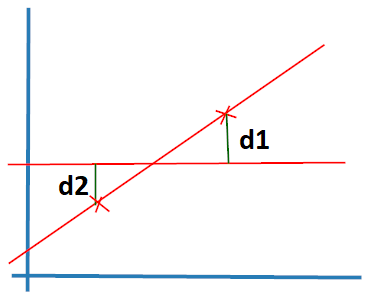# Linear Regression — Part III — R Squared

R Squared is one of the metrics by which we can find the accuracy of a model that we create.

R squared metrics works only if the regression model is linear.R-Squared: Image by AuthorPhoto by Sheri Hooley on Unsplash

SSE — Sum of Squares of Residuals (Errors)

SSR is the sum of all the difference between the original and predicted values.SSE: Image by Author

Here SSR = e1 + e2 + …. + en

SST — Sum of Squares of Total

SST is the sum of all the distances between the Y predicted values and the Y mean value.SST: Image by Author

SST = d1 + d2 + …. + dn

Now you can see that the SSR is the numerator and SST is the denominator.

If the SSR value is less than SST, then the SSR/SST value will be less than 1.

So, as the SSR value decreases, the SSR/SST value will also move closer to 0.

As R2 is 1 — (SSR/SST), the R2 value (accuracy) will be high, as much as the SSR/SST value is low.

i.e. If we summarize it, when the residuals (SSR) are less, the accuracy will be high.

# Conclusion:

R Squared is an error metrics of a Linear Regression model which is the measure of accuracy of the model.

Accuracy will be high as much as the residuals are low.

To know more about Linear Regression please check the below posts:

Please drop your ideas on error metrics in comments. It will be useful for me and all the readers!

Thank you!

Like to support? Just click the heart icon ❤️.

Happy Programming.

0